iSoul In the beginning is reality.

# Lorentz transformation derivations

What follows are four derivations of the Lorentz transformation from the complete Galilei (Galilean) transformations in space with time (3+1) and time with space (1+3). Their intersection is linear space and time (1+1), which is the focus of the derivations. The other dimensions may be reached by rotations in space or time.

I. Space with Time (3+1)

Consider two inertial frames of reference O and O′, assuming O to be at rest while O′ is moving with velocity v with respect to O in the positive x-direction. The origins of O and O′ initially coincide with each other. A light signal is emitted from the common origin and travels as a spherical wave front. Consider a point P on a spherical wavefront at a distance x and x′ from the origins of O and O′ respectively. According to the second postulate of the special theory of relativity the speed of light, c, is the same in both frames, so for the point P:

x = ct, and x′ = ct′.

A. Time velocity

Define velocity v as the time velocity vt = ds/dt. Consider the standard Galilean transformation of ct and x with a factor γ, which is to be determined and may depend on β, where β = v/c:

x′ = γ(x − vt) = γ(x − βct) = γx(1 − β).

The inverse transformation is the same except that the sign of β is reversed:

x = γ(x′ + vt′) = γ(x + βct) = γx′(1 + β).

# Light clocks with multidimensional time

A previous post on this subject is here. One reference for this post is V. A. Ugarov’s Special Theory of Relativity (Mir, 1979).

A light clock is a device with an emission-reflection-reception cycle of light that registers the current time and stance in units of cycle length and duration. Consider two identical light clocks, at first in their reference frames at rest, K, K´. Then, as the light clock in K´ moves transversely relative to K with uniform motion at velocity v (right), from K one observes the following: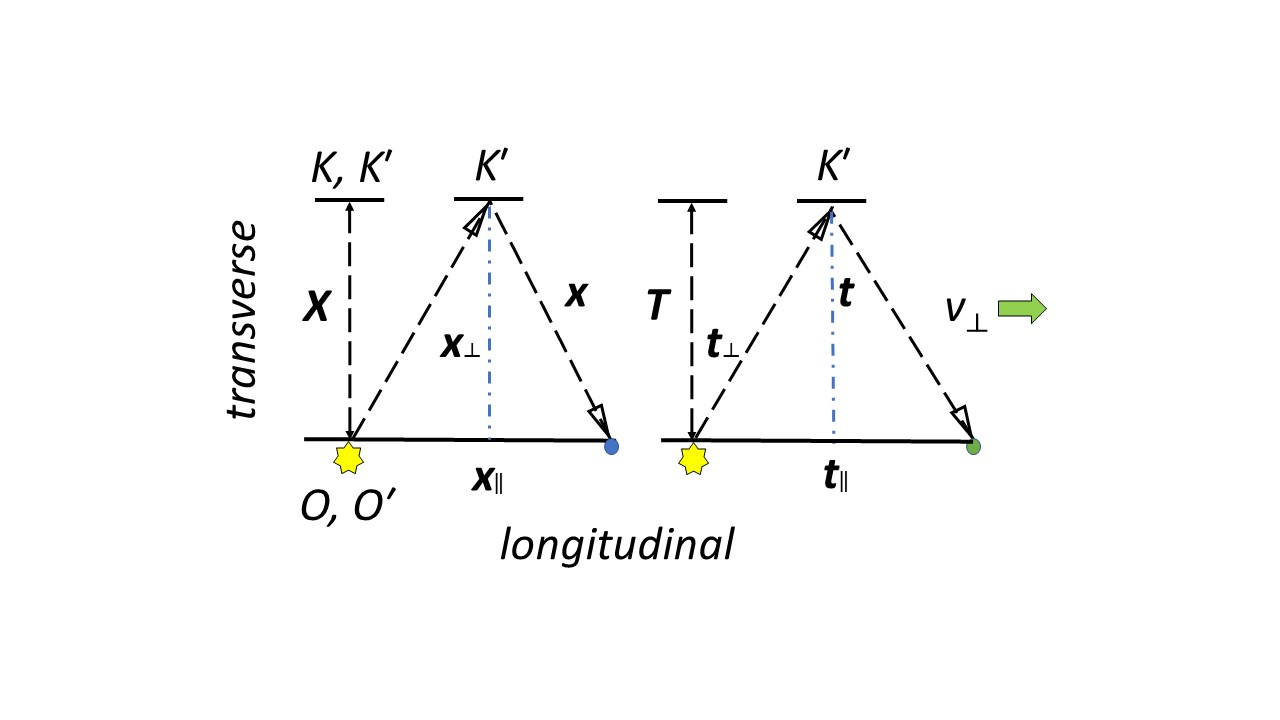The illustration above shows one cycle length of the light path (i.e., wavelength), X, on the left and one cycle duration (i.e., period), T, on the right at rest in reference frames K, K´. For the reference frame K´, in motion relative to reference frame K, call the arc length of one cycle of the light path x. Call the distance between the beginning and ending spacepoints of one cycle x. For the reference frame K´ relative to reference frame K, call the arc time of one cycle of the light path t. Call the distime between the beginning and ending timepoints of one cycle t.

# Clock-rods

A clock-rod is a linear or planar clock with a parallel rod attached to it. A mechanical or electronic clock-rod might look like this: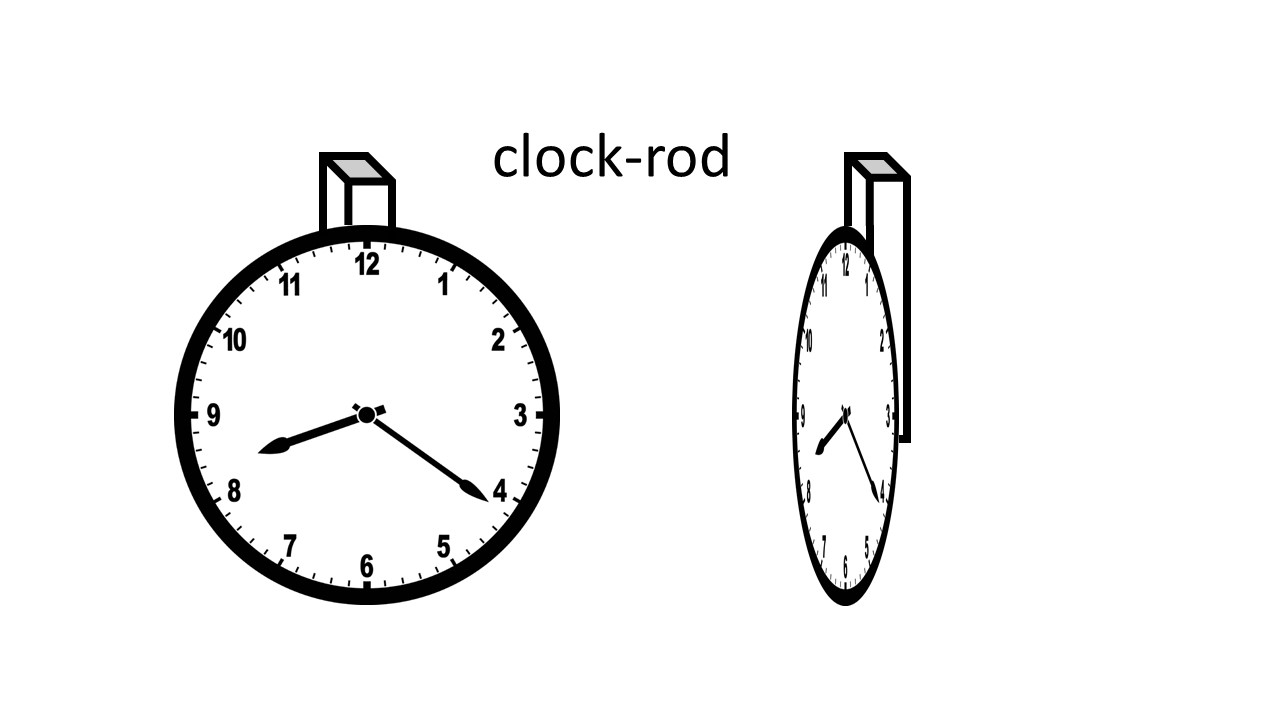Three clock-rods mutually perpendicular would measure length and duration in all directions.

A light clock-rod is conceptually like this:The clock and rod are parallel to each other so that parallel or perpendicular motion would change either the measurements of either the clock or the rod but not both. A complete harmonic cycle is not affected by motion: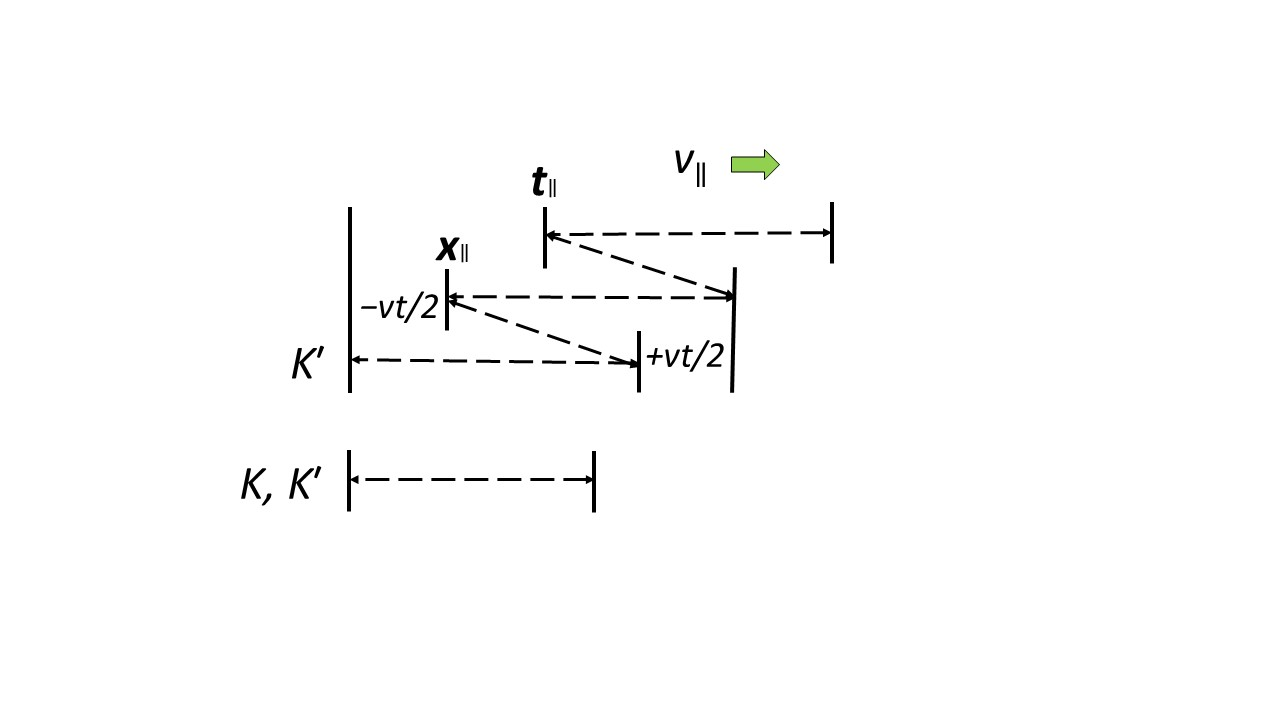The +vt/2 increased distance of the first half-cycle is offset by the −vt/2 decreased distance of the second half-cycle. Likewise for the times +2d/v and −2d/v.

# From spacetime to space and time

This relates to the post here.

There are three dimensions of motion with two measures of the extent of motion, which makes a total of six metric dimensions of motion. But these six metric dimensions collapse into two structures of one and three dimensions as the conversion factor approaches infinity.

The invariant proper length, , is:

dσ² = dr²dt²/ç² = dr1² + dr2² + dr3² – dt²/ç² = dr² – dt1²/ç² – dt2²/ç² – dt3²/ç² = dr1² + dr2² + dr3² – dt1²/ç² – dt2²/ç² – dt3²/ç².

As the conversion factor, ç, the pace of light, approaches infinity, this becomes

dσ² = dr² = dr1² + dr2² + dr3².

That is, the time coordinates separate from the invariant length, which becomes the Euclidean distance of three dimensional space. Time is left as an invariant scalar called the time.

The invariant proper time, , is:

dτ² = dσ²/c² = dr²/c² – dt² = (dr1² + dr2² + dr3²)/c² – dt² = dr²/c² – dt1² – dt2² – dt3² = (dr1² + dr2² + dr3²)/c² – dt1² – dt2² – dt3².

As the conversion factor, c, the speed of light, approaches infinity, this becomes

dτ² = – dt² = – dt² = – dt1² – dt2² – dt3².

That is, the length coordinates separate from the invariant time, which becomes the Euclidean distime of three dimensional time. Space is left as an invariant scalar called the stance.

The result is that six dimensional spacetime collapses into 3D space with scalar time or 3D time with scalar space.

# Duality as a convention

Is color an absorption phenomena or an emission phenomena? The answer is that it’s both. But absorption works subtractively whereas emission works additively. The question then is whether color is subtractive or additive. Again the answer is that it’s both. Color is a duality.

Does an artist work with subtractive colors or additive colors? Here the answer is one or the other. A painter works with pigments that are subtractive, whereas a glass artist works with stained glass that is additive. Even though absorption and emission are operating in both cases, working with color requires picking one or the other (except for mixed media).

A simultaneity convention can also be a duality. What has been called apparent simultaneity is the convention that the backward light cone is simultaneous. But it is possible to adopt a complementary convention in which the forward light cone is simultaneous (see here). Either of these is something of a combination of Newton’s and Einstein’s physics.

One could recover Newtonian physics by adopting a combination of the backward and forward light cone simultaneity conventions. For an absorption event the backward light cone is simultaneous. For an emission event the forward light cone is simultaneous. This is like half-duplex communication (push to talk, release to listen). Such a duality convention recovers Newtonian physics because it is as if the speed of light is instantaneous in all directions.

# Galileo’s reciprocity

From Galileo’s Dialogue Concerning the Two Chief World Systems, translated by Stillman Drake (UC Press, 1967):

Salv. Now imagine yourself in a boat with your eyes fixed on point of the sail yard. Do you think that because the boat is moving along briskly, you will have to move your eyes in order to keep your vision always on that point of the sail yard and to follow its motion?

Simp. I am a sure that I should not need to make any change at all; not just as to my vision, but if I had aimed a musket I should never have to move it a hairsbreadth to keep it aimed, no matter how the boat moved.

Salv. And this comes about because the motion the ship confers upon the sail yard, it confers upon you and also upon your eyes, so that you need not move them a bit in order to gaze at the top of the sail yard, which consequently appears motionless to you. [And the rays of vision go from the eye to the sail yard just as if a cord were tied between the two ends of the boat. Now a hundred cords are tied at different fixed points, each of which keeps its place whether the ship moves or remains still.] p.249-250

Galileo is portraying motion as viewed by a human observer. The implication is that the observer in another ship would be observing the same kinds of things. Then two observers in motion with respect to one another who observe one another must face one another. That is, they are positioned opposite one another, effectively each turned 180º from the other.

This is the Galileo Reciprocity Principle, the convention that an observed frame has the opposite orientation as the frame from which it is observed, which ensures that corresponding velocities are equal. It is the opposite of the Einstein Reciprocity Principle, the convention that an observed frame has the same orientation as the frame from which it is observed.

# Relativity of orientation

The Principle of Relativity states that the laws of physics are the same in all inertial frames of reference (IRF). Since a frame of reference includes an orientation, that is, a convention as to which rectilinear semi-axes are positive (and so which are negative). Since there is no preferred frame of reference, each frame has its own orientation, not the orientation of a particular frame. That means IRF orientations are what is called “body-fixed” orientations.

A frame of reference is called “body-fixed” if it is conceptually attached to a rigid body, such as a vehicle, watercraft, aircraft, or spacecraft. Body-fixed frames are inertial frames if the body to which the frame is affixed is in inertial motion. The body is usually referenced in anthropomorphic terms, such as its left, right, face, or back, although some craft have their own terms, notably, ships with port, starboard, fore, and aft.

Consider the following scenario of cars in five lanes, oriented so that their forward direction is positive, with their unsigned speeds shown relative to the two parked cars in the middle lane:Compare the direction of cars B, C1, C2, and D according to the frames attached to the five cars:

# Velocity reciprocity clarified

This is a follow-on to posts here and here.

It is common to derive the Lorentz transformation assuming velocity reciprocity, which seems to say that if a body at rest in frame of reference is observed from a frame of reference S that travels with relative velocity +v, then a body at rest in frame of reference S will be observed from the frame of reference to be traveling with velocity –v. But that’s not the case.

Consider the typical scenario in which a person standing on the earth (embankment, station) with frame of reference S observes a person sitting in a railway car with frame of reference . Say they are both waving their right hands and their frame of reference follows a right-hand orientation: the positive direction is toward their right.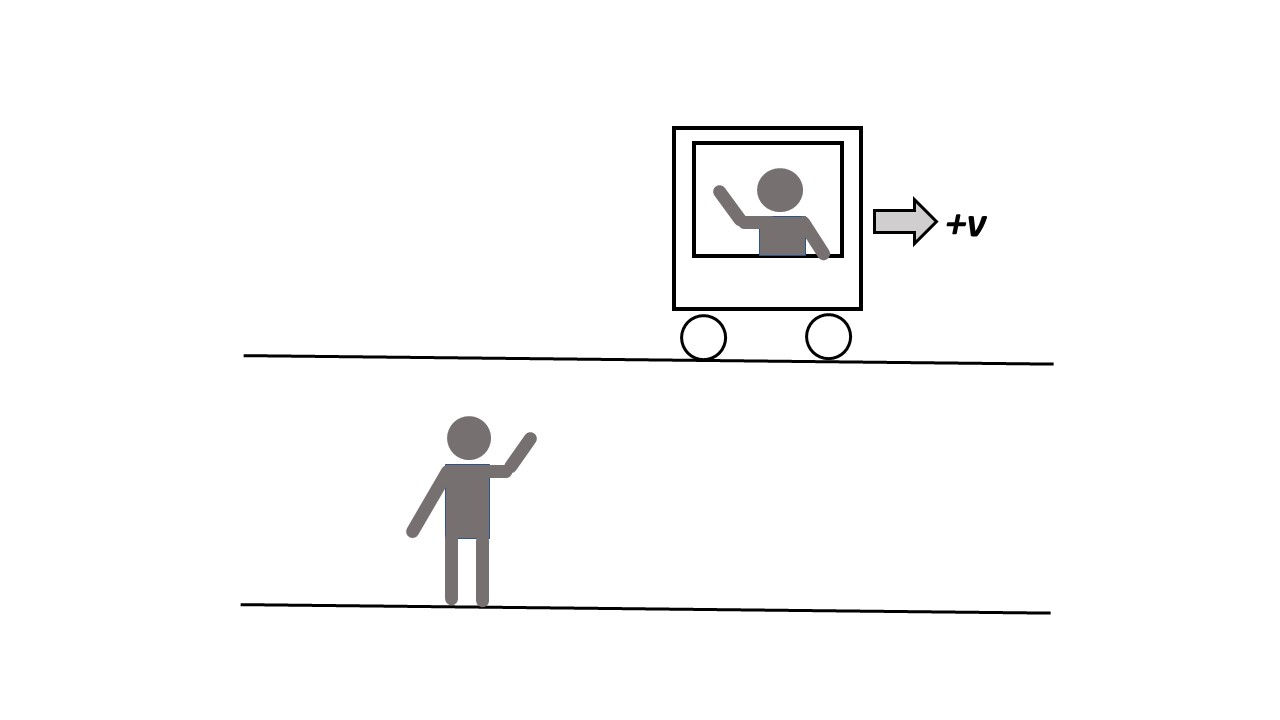The first illustration shows the scenario from behind the observer standing on the earth in frame S, who observes the passenger sitting in the train moving to their right with velocity +v. The scenario is typically presented from only this perspective, that of an observer at rest in frame A, even if the perspective of an observer at rest in frame is described.

# Galilean relativity defended

Galilean relativity is a relational theory of motion as a function of time, which leads to the Galilean transformation. Here is a defense of Galilean relativity from two postulates:

(1) The Galilean principle of relativity, which states that the laws of mechanics are invariant under a Galilean transformation.

(2) A convention that rectilinear coordinates for frames of reference follow the right-handed rule: the unit vectors i, j, and k are related as i × j = k.

The Galilean transformation for constant motion on the x axis is x´ = xvt,  and t´ = t. Postulate (2) means if the extended right-hand thumb points to the positive X axis and the extended right-hand first finger points to the positive Y axis, then the right-hand middle finger points orthogonally to the positive Z axis.

The standard configuration for derivations of the Lorentz transformation consists of two inertial frames of reference moving relative to each other at constant velocity, with Cartesian coordinates such that the x and x′ axes are collinear facing the same direction:In this case the velocity of S´ relative to S is +v and the velocity of S relative to S´ is –v. This is called the principle of velocity reciprocity.

# Two principles of velocity reciprocity

Velocity reciprocity in relativity theory is the relation between two observers, each associated with a frame of reference and moving at different, but constant, velocities. That is, an observer-frame S observes another observer-frame traveling with velocity +v relative to observer-frame S. A velocity reciprocity relation concerns the velocity of S that is observed by . Einstein’s principle of velocity reciprocity states that each velocity is the same magnitude (speed) but is in the opposite direction. That is, the velocity of S observed by  is –v.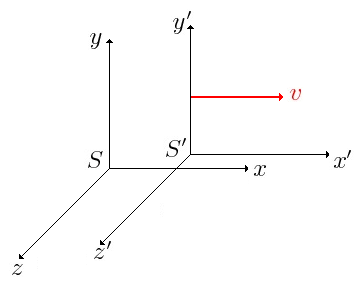Einstein’s principle of velocity reciprocity reads

We postulate that the relation between the coordinates of the two systems is linear. Then the inverse transformation is also linear and the complete non-preference of the one or the other system demands that the transformation shall be identical with the original one, except for a change of v to −v. Ref.